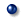Flash Physics animation

When the name below is clicked, the animation made by 'FLASH' is displayed.
Please click "Return" of a browser when it returns to this menu panel.Foil electroscope 　　　　 　The foil electroscope opens and shuts by moving the charge.Foil electroscope2 　　　 　The foil electroscope opens and shuts by moving a free electron.Electric Potential 　　　　 　It is an explanation chart where potential was shown by height.Kirchhoff's law 　　　　 　The relation between the current and potential when the switch is switched in the electric circuit where the resistance of the relation and 3,4 piece of the current and potential when two resistance is connected the series and connected parallel was used is shown.Wheatstone's bridge 　　　 　Potential at both ends of the galvanometer at the center becomes equal when the ratio of the resistance two forward is equal to the ratio of two rear side resistance, and the current doesn't flow to the galvanometer.Change in voltage of terminal by internal resistance 　　　　 　It is the one that it explained the voltage descent happens because of internal resistance when the current flows to the circuit, and the voltage of the terminal falls.Potential difference and the same electric field 　　　　 　The inclination becomes strength of the electric field from the distance and the potential difference between pole boards.Connection of capacitor 　　　 　It is the one that the relation between the charge and the potential difference saved when the capacitor is connected the series and connected parallel was shown.Connected circuit of capacitor 　　　　 　It is the one that the charge saved in connected circuit of the capacitor was shown.Resistance and connection of capacitor 　　　　 　It is the one that the relation between the charge saved when resistance is a capacitor, is connected the series, and connected parallel and the potential difference and the current was shown.Connected circuit of resistance and capacit 　　　 　It is the one that the front of savings of the charge in the capacitor of connected circuit of resistance and the capacitor and the following potential differences were shown.Movement of electron and the direction of current 　　　　 　The current seems to move to the right though the electron moves to the left.Power that current receives in magnetic field 　　　　 　It does because of the direction of the power that the direction of the current, the direction of the magnetic field, and the current receive.Direct current motor 　　　 　The motor rotates by the power that the current receives from the magnetic field.An incidence electron moves vertical to the same magnetic field. 　　　　 　An incidence electron vertically receives Lorentz force to a vertical direction to the speed and the magnetic field of the electron in the same magnetic field and equal velocity yen moves.Cyclotron 　　　　 　The proton is accelerated in the electric field, and equal velocity yen moves in the magnetic field. It is accelerated fast by the repetition.Hall effect 　　　 　The difference is caused by the difference of the supporter of the current of the semiconductor in the electric field in the semiconductor.Electromagnetic induction(Lenz's law) 　　　　 　The magnet is brought close or is kept away from the coil, and faradic flows to the coil. Animation of two kinds when rotating in case of the case to make the magnet reciprocate is moved.Dynamo 　　　　 　The electromagnetic induction happens because the magnetic field where the coil is carried out changes if the coil is rotated in the magnetic field.Induced electromotive force 　　　 　When the conductor moves in the magnetic field, a free electron in the conductor receives Lorentz force, and it collects, and, on the other hand of the conductor, the electromotive force is caused in the conductor.Pn junction 　　　　 　The hall of p type semiconductor is supplied from the plus, and the electron of n type semiconductor is supplied from the minus. It changes into heat and the energy of light if the hall knocks against the electron on the bonded surface.

Electromagnetism　　　Battery　　　Wave　　　Sound wave　　　Light Wave　　　Mechanics　　　Thermology　　　　　　　　　　　　　　　To top page.